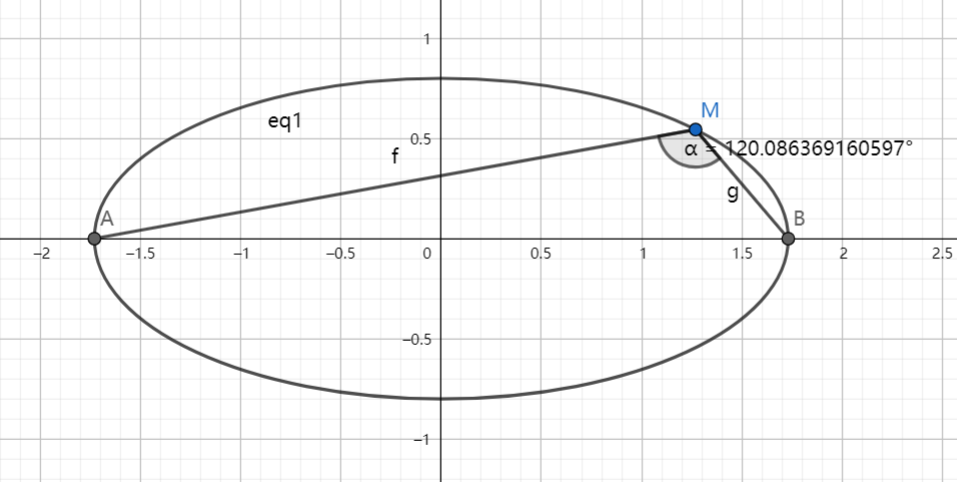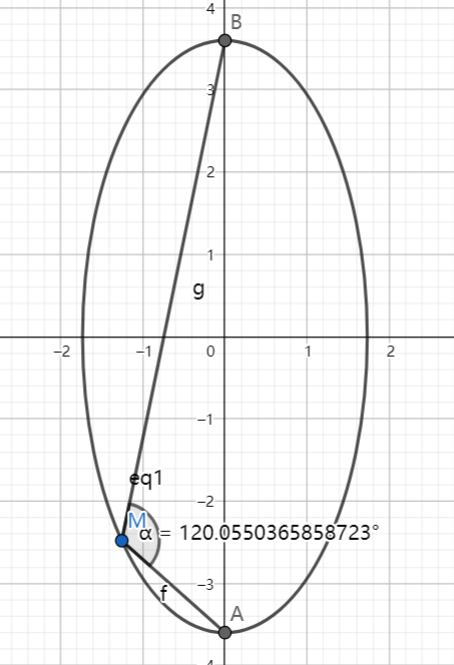# SAT1000 - P765

Geometry Level pending

Let $A,B$ be the end points of the major axis of the ellipse $C:\dfrac{x^2}{3}+\dfrac{y^2}{m}=1$.

If there exists point $M$ on the ellipse so that $\angle AMB = \dfrac{2\pi}{3}$, find the range of $m$.

These pictures show the two cases:Have a look at my problem set: SAT 1000 problems

×